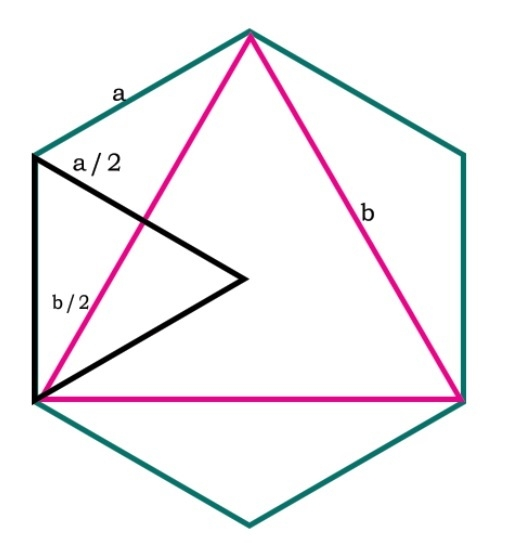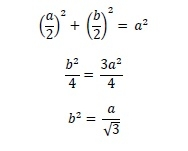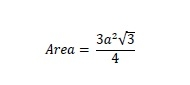# Area of the Largest Triangle inscribed in a Hexagon in C++

Here we will see the area of largest triangle which is inscribed in regular hexagon. Each side of the hexagon is ‘a’, and each side of the triangle is ‘b’.From this diagram we can see that if we make one triangle using one side of hexagon, then these two triangles are making each sides into two parts. We can see two right angled triangles also. From the Pythagorus formula, we can say that −So the area is −## Example

#include <iostream>
#include <cmath>
using namespace std;
float area(float a) {
if (a < 0 ) //if value is negative it is invalid
return -1;
float area = (3 * sqrt(3) * pow(a, 2)) / 4;
return area;
}
int main() {
float a = 6;
cout << "Area : " << area(a);
}

## Output

Area : 46.7654